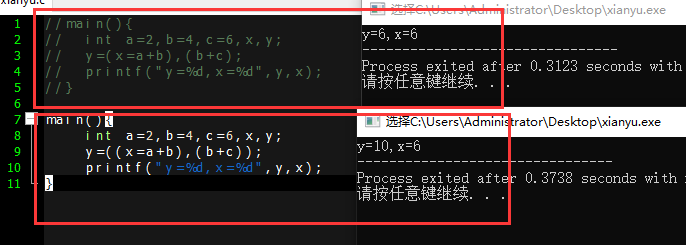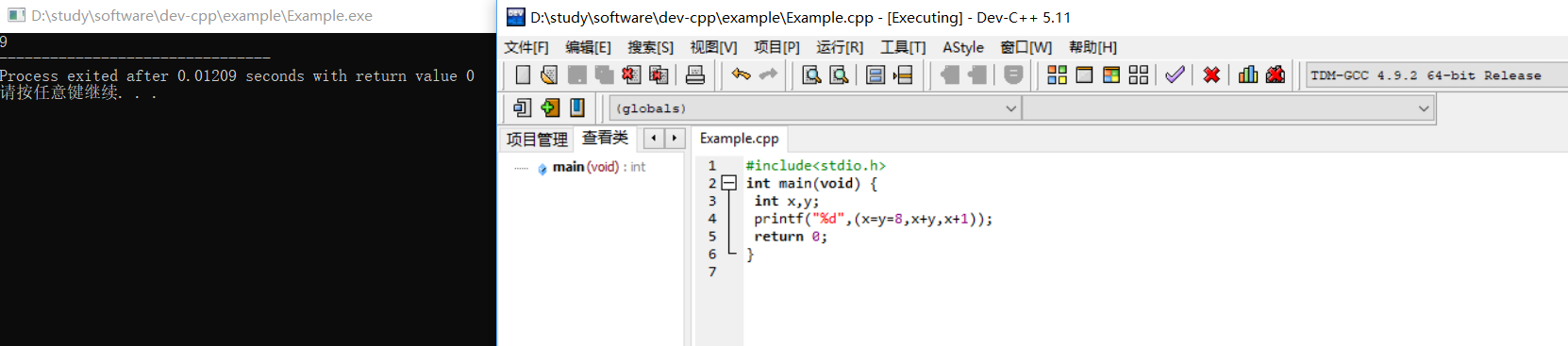• 文章目录概述:实例: 概述: 逗号运算符表达式取最后一个值返回。 特点: 优先级别最低; 自左往右执行表达式; 返回值为表达式最后一个. 实例: int i = 0; printf("%d\n", (i = 3*3 , i*5));...C语言逗号运算符 ...
文章目录概述:实例:
概述:

逗号运算符表达式取最后一个值返回。

特点:

优先级别最低;
自左往右执行表达式;
返回值为表达式最后一个.

实例:
int i = 0;
printf("%d\n", (i = 3*3 , i*5));


输出: 45.

赋值运算符优先级高于逗号运算符;
先执行i=3*3后再执行i*5;
输出:i*5的值.

详情,参见:
C语言逗号运算符


展开全文• 跟大家讲解下有关c语言逗号运算符怎么使用，相信小伙伴们对这个话题应该也很关注吧，现在就为小伙伴们说说c语言逗号运算符怎么使用，小编也收集到了有关c语言逗号运算符怎么使用的相关资料，希望大家看到了会喜欢。...
跟大家讲解下有关c语言逗号运算符怎么使用，相信小伙伴们对这个话题应该也很关注吧，现在就为小伙伴们说说c语言逗号运算符怎么使用，小编也收集到了有关c语言逗号运算符怎么使用的相关资料，希望大家看到了会喜欢。c语言逗号运算符的使用方法：当顺序点用结合顺序是从左至右用来顺序求值完毕之后整个表达式的值是最后一个表达式的值。本文操作环境：Windows7系统Dell G3电脑。c语言逗号运算符的使用方法：一、用法：1、当顺序点用结合顺序是从左至右用来顺序求值完毕之后整个表达式的值是最后一个表达式的值。main(){int a,s,d;s=2;d=3;a=12+(s+2,d+4);}先算括号内的值：s+2=4d+4=7；括号内应为(4,7)括号内取值只取最后一个如果没有括号则取第一个；a=12+7=19。x=(y=3,(z = ++y+2) +5);是首先把y赋值为3,把y递增为4,然后把4加上2,把结果6赋值给z,接下来把z加5最后把x赋为结果值11。2、注意事项：逗号运算符( , )是C语言运算符中优先级最低的一种运算符。二、其它用法：1、在for里面的应用：int i;int j;for(i=0,j=0;i<5;i++,j++)2、当分隔符：int i,j；相关学习推荐：C语言教程视频以上就是c语言逗号运算符怎么使用的详细内容！来源：php中文网郑重声明：本文版权归原作者所有，转载文章仅为传播更多信息之目的，如作者信息标记有误，请第一时候联系我们修改或删除，多谢。
展开全文• C语言逗号运算符和逗号表达式C语言逗号运算符和逗号表达式y = (x = a + b), (b + c)结果奇怪 C语言逗号运算符和逗号表达式 在Ｃ语言中逗号“，”也是一种运算符，称为逗号运算符。 其功能是把两个表达式连接 其一般...
C语言逗号运算符和逗号表达式C语言逗号运算符和逗号表达式y = (x = a + b), (b + c)结果奇怪
C语言逗号运算符和逗号表达式
在Ｃ语言中逗号“，”也是一种运算符，称为逗号运算符。 其功能是把两个表达式连接
其一般形式为：
表达式 1，表达式 2

1+1，2+2

其求值过程是分别求两个表达式的值，并以表达式 2 的值作为整个逗号表达式的值。
main(){
int a=2,b=4,c=6,x,y;
y=(x=a+b),(b+c);
printf("y=%d,x=%d",y,x);
}

本例中，y 等于整个逗号表达式的值，也就是表达式 2 的值，x 是第一个表达式的值。对于逗号表达式还要说明两点：

逗号表达式一般形式中的表达式 1 和表达式 2 也可以又是逗号表达式。
例如：

表达式 1，(表达式 2，表达式 3)

形成了嵌套情形。因此可以把逗号表达式扩展为以下形式：
表达式 1，表达式 2，…表达式 n

整个逗号表达式的值等于表达式 n 的值。

程序中使用逗号表达式，通常是要分别求逗号表达式内各表达式的值，并不一定要求整个逗号表达式的值。
并不是在所有出现逗号的地方都组成逗号表达式，如在变量说明中，函数参数表中逗号只是用作各变量之间的间隔符.

y = (x = a + b), (b + c)结果奇怪
逗号运算符是所有运算符中级别最低的。因此，下面两个表达式的作用是不同的:
int a=2,b=4,c=6,x,y;
y=(x=a+b),(b+c);  x=6 ;y=6
y=((x=a+b),(b+c);  x=6 y=10


逗号运算符的优先级最低
所以
y=(x=a+b),c+d;
先执行x=a+b
然后执行y=x
c+d没意义 最终y=6
如果为y=((x=a+b),(b+c)
那么意思就是全算完在赋值
即y=c+d了展开全文• 引入 设以下变量均为 int 类型，表达式的值不为 9 的...这个逗号运算符java里面还像没有，它的逗号是分割符。c有 拿(x=y=8,x+y,x+1)他求的是最后一个逗号后的值为9 在有的地方它不是当逗号运算符用，当分隔符用 ...
引入
设以下变量均为 int 类型，表达式的值不为 9 的是 C
A． (x=y=8,x+y,x+1) B． (x=y=8,x+y,y+1) C． (x=8,x+1,y=8,x+y) D． (y=8,y+1,x=y,x+1)

运算
这个逗号运算符java里面还像没有，它的逗号是分割符。c有
拿(x=y=8,x+y,x+1)他求的是最后一个逗号后的值为9
在有的地方它不是当逗号运算符用，当分隔符用展开全文• 简要解释一下C语言逗号运算符在一些工作场景下的作用 Thanks♪(･ω･)ﾉ有问必答
• 概念：逗号运算符，多个表达式可以用逗号分开，但整个表达式的值是最后一个表达式的值。平时在写程序的时候，我们常用while，for，但是逗号运算符是for的一种扩展，以便在循环的开头包含更多的表达式。下面就以案例...
• C语言逗号运算符（,）是优先级最低的运算符。 所谓的优先级是指当有许多运算符在一起的时候，谁先执行的问题。例如：1+2*3，先执行加号运算符还是先执行乘号运算符。 赋值运算符（=）是优先级较低的运算符，但...
• 前言：逗号运算符逗号运算符是单目运算符，其功能是把两个表达式组成一个表达式，分别求表达式的值，最后一个表达式的值作为整个表达式的值。代码源码：#include void douhao(void);void main(void){printf("hello......

# c语言逗号运算符c语言 订阅